﻿ 湖南省城乡居民个人医疗负担预测研究

湖南省城乡居民个人医疗负担预测研究Study on Prediction of Individual Medical Burden of Urban and Rural Residents in Hunan Province

Abstract: Objective: To evaluate the changing trend of the medical economic burden of personal health expenditures of urban and rural residents in Hunan Province, to provide a reasonable reference for the health department to formulate and adjust medical and health policies. Methods: The GM(1,1) model was used to predict the personal health expenditures of urban and rural residents in Hunan Province from 2019 to 2023 and their proportion in total health expenditures. Results: It is predicted that from 2019 to 2023, the proportion of personal health expenditures of urban and rural residents in Hunan Province in total health expenditures will continue to decline, which will drop from 30.20% to 25.63%, a decrease of 4.57%. The annual decrease of 1.14% indicates that the medical economic burden of personal health expenditures for urban and rural residents in Hunan Province will gradually be reduced, the probability of “poverty due to illness and return to poverty due to illness” will gradually decrease, and the fairness of its health financing structure will be further improved Get optimized. Conclusion: It is recommended that the government scientifically increase financial investment and adjust the internal structure of government health expenditures; actively support social health expenditures and reasonably increase the proportion of social medical insurance reimbursement; actively support the development of commercial health insurance.

1. 资料来源与方法

1.1. 资料来源

1.2. 研究方法

1.3. 统计学方法

2. 结果

2.1. 基于GM(1,1)模型的个人卫生支出占卫生总费用比例预测Table 1. Personal health expenditure accounts for the proportion of total health expenses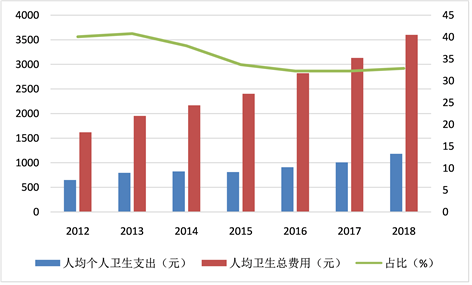Figure 1. Proportion of per capita health expenses of urban and rural residents in Hunan Province from 2012 to 2018

2.2. 级比检验，建模可行性分析

${X}^{\left(0\right)}=\left({X}^{\left(0\right)}\left(1\right),{X}^{\left(0\right)}\left(2\right),\cdots ,{X}^{\left(0\right)}\left(7\right)\right)=\left(649.72,796.86,824.49,809.97,910.04,1009.45,1183.00\right)$ ；其次，求级比值； $\sigma =\left(0.8154,0.9665,1.0179,0.8900,0.9015,0.8533\right)$，发现所有的级比值都恰好在区间(0.778800783, 1.284025417)内，因此模型的建立能进行。

2.3. 灰色GM(1,1)模型的建立

${X}^{\left(1\right)}=\left({X}^{\left(1\right)}\left(1\right),{X}^{\left(1\right)}\left(2\right),\cdots ,{X}^{\left(1\right)}\left(7\right)\right)=\left(649.72,1446.58,2271.07,3081.04,3991.08,5000.53,6183.53\right)$。其次，构造均值数列，即

${Z}^{\left(1\right)}=\left({Z}^{\left(1\right)}\left(2\right),\cdots ,{Z}^{\left(1\right)}\left(7\right)\right)=\left(1048.15,1858.825,2676.055,3536.06,4495.805,5592.03\right)$

$Z=\left(\begin{array}{c}\frac{1}{2}\left[{X}^{\left(1\right)}\left(1\right)+{X}^{\left(1\right)}\left(2\right)\right]\\ \frac{1}{2}\left[{X}^{\left(1\right)}\left(2\right)+{X}^{\left(1\right)}\left(3\right)\right]\\ \frac{1}{2}\left[{X}^{\left(1\right)}\left(3\right)+{X}^{\left(1\right)}\left(4\right)\right]\\ \frac{1}{2}\left[{X}^{\left(1\right)}\left(4\right)+{X}^{\left(1\right)}\left(5\right)\right]\\ \frac{1}{2}\left[{X}^{\left(1\right)}\left(5\right)+{X}^{\left(1\right)}\left(6\right)\right]\\ \frac{1}{2}\left[{X}^{\left(1\right)}\left(6\right)+{X}^{\left(1\right)}\left(7\right)\right]\end{array}\right)=\left(\begin{array}{c}1048.15\\ 1858.825\\ 2676.055\\ 3536.06\\ 4495.805\\ 5592.03\end{array}\right)$

$Y=\left(\begin{array}{c}{X}^{\left(0\right)}\left(2\right)\\ {X}^{\left(0\right)}\left(3\right)\\ {X}^{\left(0\right)}\left(4\right)\\ {X}^{\left(0\right)}\left(5\right)\\ {X}^{\left(0\right)}\left(6\right)\\ {X}^{\left(0\right)}\left(7\right)\end{array}\right)=\left(\begin{array}{c}796.86\\ 824.49\\ 809.97\\ 910.04\\ 1009.45\\ 1183\end{array}\right)$

$B=\left(\begin{array}{c}-{Z}^{\left(1\right)}\left(2\right)\\ -{Z}^{\left(1\right)}\left(3\right)\\ -{Z}^{\left(1\right)}\left(4\right)\\ -{Z}^{\left(1\right)}\left(5\right)\\ -{Z}^{\left(1\right)}\left(6\right)\\ -{Z}^{\left(1\right)}\left(7\right)\end{array}\right)=\left(\begin{array}{cc}-1048.15& 1\\ -1858.825& 1\\ -2676.055& 1\\ -3536.06& 1\\ -4495.805& 1\\ -5592.03& 1\end{array}\right)$

${\Lambda }_{P}={\left({\Lambda }_{a},{\Lambda }_{b}\right)}^{\text{T}}={\left({B}^{\text{T}}B\right)}^{-1}{B}^{\text{T}}Y=\left(\begin{array}{c}-0.08387\\ 653.83286\end{array}\right)$，由此可得： $a=-0.08387$$b=653.83286$

$\frac{b}{a}=-7796.137513$${X}^{\left(0\right)}\left(1\right)-\frac{b}{a}=8445.857513$。最后，解得湖南省城乡人均个人卫生费用支出的预测模型为： ${\Lambda }_{{X}^{\left(1\right)}\left(k+1\right)}=8445.857513{\text{e}}^{0.08387k}-7796.137513$，通过累减还原得到预测的值。

${X}^{\left(0\right)}\left(1\right)-\frac{b}{a}=14377.12389$。因此，最终可以得到其GM(1,1)模型为：

${\Lambda }_{{X}^{\left(1\right)}\left(k+1\right)}=14377.12389{\text{e}}^{0.12489k}-12756.86389$，通过累减还原得到预测的值。

2.4. 模型的拟合检验

GM(1,1)模型需要精度合适才能用以对未来的预测。通常，为了检验预测模型是不是可靠，常用后验差比值法。Table 2. Model accuracy level evaluation standard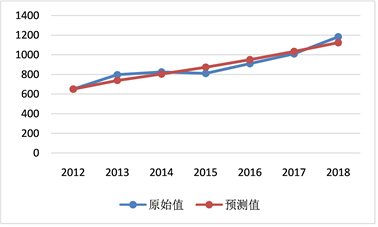Figure 2. Per capita personal health expenditure fitting effect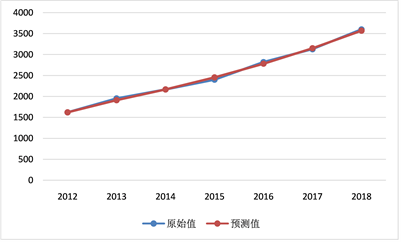Figure 3. Fitting effect of per capita total health expenditure

2.5. 对2019年~2023年湖南省人均个人卫生支出进行预测Table 3. Predicted value of per capita personal health expenditure and its proportion in total health expenditure from 2019 to 2023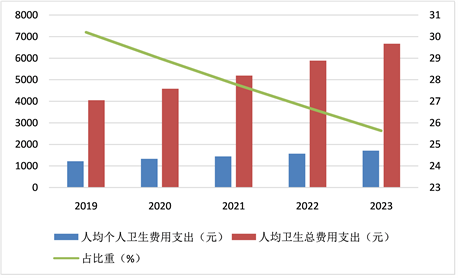Figure 4. Per capital personal health expenditure and accounting map of Hunan Province 2019-2023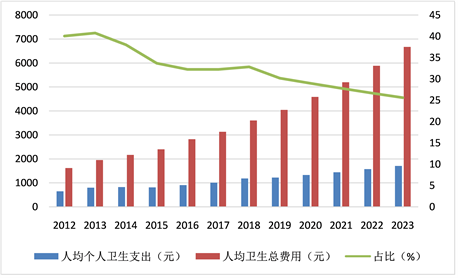Figure 5. Per capita personal health expenditure and its proportion in Hunan Province from 2012 to 2023

3. 讨论与结论

3.1 明确各类医疗机构的功能定位

3.2 提升基层医疗机构的医疗服务水平

3.3 合理分配医疗保险报销比例

 邵昆. 浅谈就医顾客需求与行为[J]. 现代医院管理, 2007, 10(5): 38-40.

 周连红. 社区卫生服务中心的定位和发展方向[J]. 中华实用医药, 2004, 4(9): 135-136.

 孙艳华. 门诊患者选择医院的参考因素的研究[J]. 求医问药, 2012, 10(1): 327-328.

Top